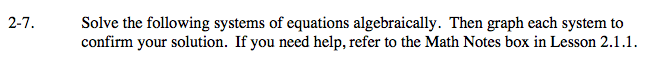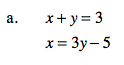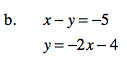Home > A2C > Chapter 2 > Lesson 2.1.1 > Problem2-7

2-7.
1. Solve the following systems of equations algebraically. Then graph each system to confirm your solution. If you need help, refer to the Math Notes box in Lesson 2.1.1. 2-7 HW eTool (Desmos). Homework Help ✎

1. x + y = 3
x = 3y − 5

2. x − y = − 5
y = −2x − 4Replace x with 3y − 5.

Solve for y.

Solve for x.Replace y with −2x − 4.

Solve for x.

Solve for y.

In the eTool below type points into the tables to explore where the two graphs intersect.
Click on the link at right for the full eTool version: A2C 2-7 HW eTool